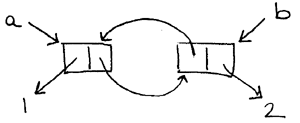```recitation 18: review for quiz 2

========================================================================

Memoization is a clever idea that allows us to save on computation. It
works by keeping track of evaluation of a procedure on a specific
argument, and simply returns the remembered value if the procedure has
already been run on that argument.

(define (memoize proc)
(let ((cache '()))
(lambda (arg)
(if (assq arg cache)
(set! cache (cons (list arg answer) cache))

(define my-sq (memoize (lambda (x) (* x x))))
(my-sq 5)

Finish the environment diagram:
``````To what does the environment pointer of P1 point?
To what does the environment pointer of P2 point?
To what does the environment pointer of P3 point?
To what does the environment pointer of P4 point?
To what does the environment pointer of P5 point?

What is the enclosing environment for E1?
What is the enclosing environment for E2?
What is the enclosing environment for E3?
What is the enclosing environment for E4?
What is the enclosing environment for E5?

To what is the variable memoize in the global environment bound?
To what is the variable my-sq in the global environment bound?
To what is the variable cache in E1 bound?
To what is the variable arg in E2 bound?

What variable is bound to the procedure object P1?
Give both the name and the environment.
What variable is bound to the procedure object P5?
Give both the name and the environment.

GE, E1, GE, E4, E2,
E4, E1, E2, GE, GE,
P3, P2, ((5 25)), 5,
proc in E4, nothing

========================================================================

* let's write insertion sort:

(define (make-sorted-list)
(cons 'sorted '()))

(define (get-data sorted-list)
(if (eq? (car sorted-list) 'sorted)
(cdr sorted-list)
(error "not a sorted-list")))

(define (insert sorted-list element)
YOUR-CODE-HERE )

(define lst (make-sorted-list))
(get-data lst)  ->  ()
(insert lst 4)
(insert lst 1)
(get-data lst)  ->  (1 4)
(insert lst 5)
(insert lst 6)
(insert lst 7)
(insert lst 3)
(get-data lst)  ->  (1 3 4 5 6 7)

* whats the order of growth?

(define (insert sorted-list element)
(cond ((null? (cdr sorted-list))
(set-cdr! sorted-list (cons element nil)))
(set-cdr! sorted-list (cons element (cdr sorted-list))))
(else (insert (cdr sorted-list) element))))

Order of growth: O(n^2)

========================================================================

* say we know that often the elements are often inserted in clumps
that are already sorted (either increasing or decreasing)

Instead of just a regular list with a pointer to the next element,
there are also pointers to the previous element.  This requires alot
more work to maintain a consistent data structure.  Here are some
helper functions for one possible implementation:

(cons element (cons nil nil)))

and a sorting routine based on this could look like:

(define (make-sorted-list)
(cons 'sorted nil))

(define (get-data sorted-list)
nil
(if (null? (cdr sorted-list))
nil
(extract (find-front (cdr sorted-list))))))

(define (insert sorted-list element)
(let ((current (cdr sorted-list))
(cond ((null? current)
;; this is the first element
(set-cdr! sorted-list new-unit))
((and (< element (get-element current))
(null? (get-prev current)))
;; add it to the front
(set-next! new-unit current)
(set-prev! current new-unit)
(set-cdr! sorted-list new-unit))
((and (>= element (get-element current))
(null? (get-next current)))
;; add it to the end
(set-prev! new-unit current)
(set-next! current new-unit)
(set-cdr! sorted-list new-unit))
((and (< element (get-element current))
(>= element (get-element (get-prev current))))
;; add the element just in front of the current element
(set-prev! new-unit (get-prev current))
(set-next! new-unit current)
(set-next! (get-prev current) new-unit)
(set-prev! current new-unit)
(set-cdr! sorted-list new-unit))
((< element (get-element current))
;; shift the current pointer to the prev element
(set-cdr! sorted-list (get-prev current))
(insert sorted-list element))
(else
;; shift the current pointer to the next element
(set-cdr! sorted-list (get-next current))
(insert sorted-list element)))))

* discuss the expected performance of the new insertion sort
implementation given our assumption about the input (that it
comes in sorted clumps)

since most of the elements will be inserted right next to the current
element, most inserts will be constant time, and the sort will be O(n).
[ a more rigorous analysis of this problem would use the term "amortized"
which you'll learn about in an algorithms course. ]

========================================================================

what code made this environment diagram?  (some parts may not be drawn)
``````
(define foo
(let ((bar nil))
(set! bar (lambda (x) (bar x)))
bar))

OR

(define foo
(let ()
(define bar (lambda (x) (bar x)))
bar))

what code made this cons cell structure?
``````
(define a (cons 1 (cons nil 2))
(define b (cdr a))
(set-car! b a)

========================================================================
```